Tom Kelliher, CS26

Sept. 5, 1996

# Performance

For a single program, here's the fundamental equation: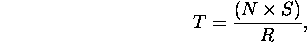where T is execution time, N is the number of machine instructions to be executed, S is the average number of clock cycles per machine instruction, and R is the clock frequency.

What can we do to improve T?

1. Bits, bytes, words.
2. k address lines yieldaddressable locations, numbered.

Little-endian byte numbering: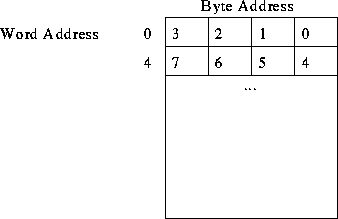Big-endian byte numbering:## Number Representation

1. Assume 32 bits/word.
2. Bit 0 is lsb; bit 31 is msb.
3. Sign-magnitude representation: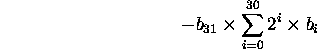4. Two's complement representation: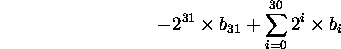## Character Representation

• ASCII, EBCDIC.
• One character per byte.

## Instruction Representation

Consider the MIPS instruction

```add \$16, \$17, \$18   # R16 = R17 + R18
```
Here's how the instruction is stored in memory: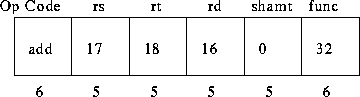# Instructions and Instruction Sequencing

Instruction types:

2. Arithmetic and logic.
3. Branch and control.
4. I/O.

Instruction set design choices:

1. Number of addresses: 3, 2, 1, 0.
3. Fixed vs. variable size instructions, fields
4. Number of general purpose registers
All affect size of an instruction.

Registers --- the programmer's ``manual cache.''

Consider the C code:

```a = b + c;
```
```add a, b, c

store r1, c
```
```move a, c
```
One address (implied operand --- accumulator):
```load a
store c
```
```push a
push b
pop c
```

## Straight Line Execution

Simple instruction cycle:

• Instruction fetch: fetch instruction pointed to by PC, update PC (How much?).
• Instruction execute.

## Branching

How do you structure a program to add n numbers?

Pseudo C code:

```i = n;
sum = 0;
while (i > 0)
sum += number to be added;
```

Pseudo assembly:

```         move n, r1
clear r0
decrement r1
branch >0, loop
move r0, sum
```
n, sum are memory locations.

How does this fit in memory and get executed?

## Condition Codes

• N, negative --- set if result is negative.
• Z, zero --- set if result is zero.
• V, overflow --- set if operation overflowed.
• C, carry --- set if carry from msb.
Meaning?

Choices:

1. Condition codes statically set by most/all instructions.
2. No condition codes.
3. Bit in instruction enables setting conditions codes (dynamic).

Effects on performance?

Effective address (EA): final location of the operand.

## Register Mode

EA is a register, specified in instruction.

```add r0, r1
```

## Absolute Mode

EA is a memory location, address specified in instruction.

```add data, sum
```

## Immediate Mode

EA is the instruction --- operand is part of the instruction.

```move #0, r0
sub #1, r1
```

## Indirect Mode

EA is memory location pointed to by register or memory word indicated in instruction.

```add (r1), r0
```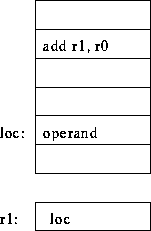## Indexed Mode

EA is memory location pointed to by sum of register and a constant (both specified in instruction).

```add 12(r0), r1
```
Sometimes called base, displacement (offset) addressing.

Not really used for what you think.

## Autoincrement, Autodecrement Modes

Stack operations: post-increment, pre-decrement.

Thomas P. Kelliher
Wed Sep 4 16:56:40 EDT 1996
Tom Kelliher